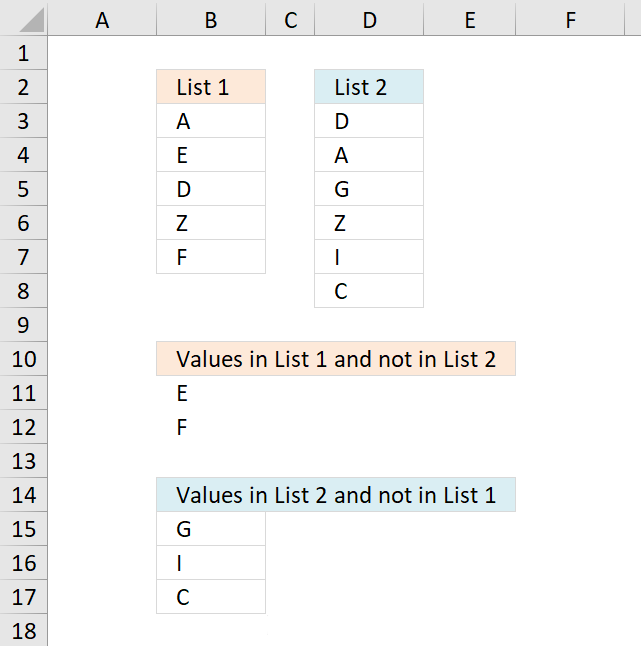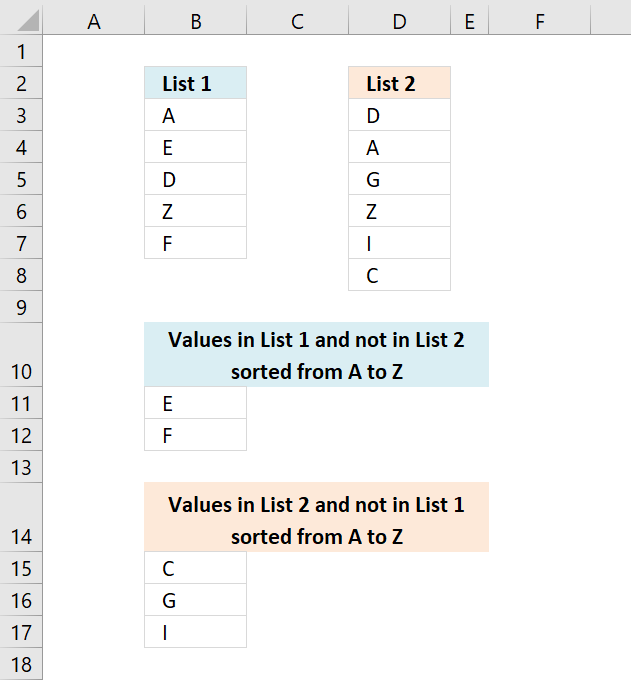Author: Oscar Cronquist Article last updated on January 05, 2019The image above demonstrates an array formula in cell B11 that extracts values that only exist in List 1 (B3:B7) and not in List 2 (D3:D8). The same formula is used in cell B15, however, with different cell references. This time it extracts values that only exist in List 2 (D3:D8).

Array Formula in B11:

=INDEX(\$B\$3:\$B\$7, SMALL(IF(COUNTIF(\$D\$3:\$D\$8, \$B\$3:\$B\$7)=0, MATCH(ROW(\$B\$3:\$B\$7), ROW(\$B\$3:\$B\$7)), ""), ROWS(\$A\$1:A1)))

To enter an array formula press and hold CTRL + SHIFT simultaneously, then press Enter once. Release all keys.

The formula bar now shows the formula enclosed with curly brackets telling you that you entered the formula successfully. Don't enter the curly brackets yourself.

Copy cell D2 and paste it down as far as needed.

Array Formula in B15:

=INDEX(\$D\$3:\$D\$8, SMALL(IF(COUNTIF(\$B\$3:\$B\$7, \$D\$3:\$D\$8)=0, MATCH(ROW(\$D\$3:\$D\$8), ROW(\$D\$3:\$D\$8)),""), ROWS(\$A\$1:A1)))

Copy cell D9 and paste it down as far as needed.

### Explaining formula in cell B11

#### Step 1 - Count values in List 1 based on values in List 1

The COUNTIF function counts values based on a condition or criteria.

COUNTIF(\$D\$3:\$D\$8, \$B\$3:\$B\$7)=0

becomes

COUNTIF({"D"; "A"; "G"; "Z"; "I"; "C"}, {"A"; "E"; "D"; "Z"; "F"})=0

becomes

{1;0;1;1;0}=0

The logical expression returns TRUE if a value in the array is equal to 0 (zero).

{1;0;1;1;0}=0

returns {FALSE; TRUE; FALSE; FALSE; TRUE}

#### Step 2 - Replace TRUE with corresponding row number

The IF function has three arguments, the first one must be a logical expression. If the expression evaluates to TRUE then one thing happens (argument 2) and if FALSE another thing happens (argument 3).

IF(COUNTIF(\$D\$3:\$D\$8, \$B\$3:\$B\$7)=0, MATCH(ROW(\$B\$3:\$B\$7), ROW(\$B\$3:\$B\$7)), "")

becomes

IF({FALSE; TRUE; FALSE; FALSE; TRUE}, MATCH(ROW(\$B\$3:\$B\$7), ROW(\$B\$3:\$B\$7)), "")

becomes

IF({FALSE; TRUE; FALSE; FALSE; TRUE}, {1; 2; 3; 4; 5}, "")

and returns

{""; 2; ""; ""; 5}

#### Step 3 - Extract k-th smallest row number

To be able to return a new value in a cell each I use the SMALL function to filter row numbers from smallest to largest.

The ROWS function keeps track of the numbers based on an expanding cell reference. It will expand automatically when the cell is copied to the cells below.

SMALL(IF(COUNTIF(\$B\$3:\$B\$7, \$D\$3:\$D\$8)=0, MATCH(ROW(\$D\$3:\$D\$8), ROW(\$D\$3:\$D\$8)),""), ROWS(\$A\$1:A1))

becomes

SMALL({""; 2; ""; ""; 5}, ROWS(\$A\$1:A1))

becomes

SMALL({""; 2; ""; ""; 5}, 1)

and returns 2.

#### Step 4 -

The INDEX function returns a value based on a cell reference and a row number (also a column number if needed).

INDEX(\$D\$3:\$D\$8, SMALL(IF(COUNTIF(\$B\$3:\$B\$7, \$D\$3:\$D\$8)=0, MATCH(ROW(\$D\$3:\$D\$8), ROW(\$D\$3:\$D\$8)),""), ROWS(\$A\$1:A1)))

becomes

INDEX(\$D\$3:\$D\$8, 2)

and returns "E" in cell B11.

### Sorted from A to ZArray Formula in B11:

=INDEX(\$B\$3:\$B\$7, MATCH(SMALL(IF((COUNTIF(\$F\$2:F2, \$B\$3:\$B\$7)+COUNTIF(\$D\$3:\$D\$8, \$B\$3:\$B\$7))=0, COUNTIF(\$B\$3:\$B\$7, "<"&\$B\$3:\$B\$7)), 1), COUNTIF(\$B\$3:\$B\$7, "<"&\$B\$3:\$B\$7), 0))

Array Formula in B15:

=INDEX(\$D\$3:\$D\$8, MATCH(SMALL(IF((COUNTIF(\$F\$9:F9, \$D\$3:\$D\$8)+COUNTIF(\$B\$3:\$B\$7, \$D\$3:\$D\$8))=0, COUNTIF(\$D\$3:\$D\$8, "<"&\$D\$3:\$D\$8)), 1), COUNTIF(\$D\$3:\$D\$8, "<"&\$D\$3:\$D\$8), 0))

### Get the Excel fileCompare-and-filter-values-in-two-lists-1.xlsx